Germán Rodríguez
Multilevel Models Princeton University## A Growth Curve Model

We will replicate the analysis in Goldstein (1995), Sections 6.4 and 6.5 starting on page 91, dealing with the height of school boys measured on nine occassions between ages 11 and 13. An analysis using MLwiN macros is available here, but we will use Stata and R.

### The Data

The data are available on this website as `oxboys.dta`. We read the data and plot the individual growth curves. Note that age is centered at 12.25.

```. use https://grodri.github.io/datasets/oxboys.dta, clear
(Data from Goldstein (1995) on children's height on nine occasions)

. twoway  line height age, connect(ascending) color(gs12) ///
>   || scatter height age, color(black) legend(off) ///
>   title(Height of 26 boys at ages 11 to 13) ///
>   xt(Age (centered at 12.25)) yt(Height (cm))

. graph export oxboys.png, width(500) replace
file oxboys.png saved as PNG format
```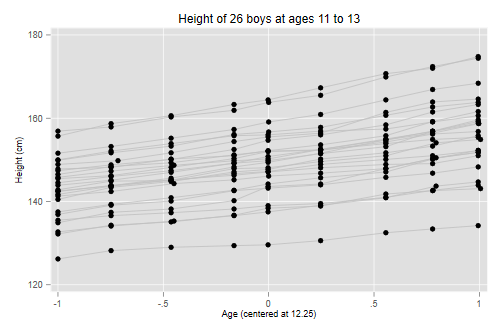```> library(haven)
> library(ggplot2)
> library(dplyr)
> ggplot(group_by(oxboys, id), aes(age, height, group=id)) +
+   geom_line(color="#c0c0c0") + geom_point() +
+   ggtitle("Height of 26 boys at ages 11 to 13")
> ggsave("oxboysr.png", width = 500/72, height = 400/72, dpi = 72)
```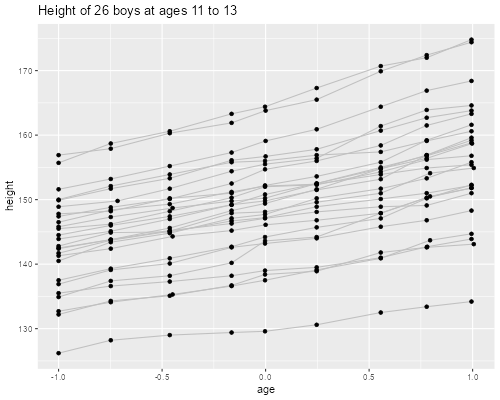### The Basic Model

The basic model used by Goldstein is a fourth-degree polynomial on age, where the constant, linear and quadratic coefficients are random at the child level with an unstructured variance-covariance matrix.

To this model we add a fixed seasonality component based on the cosine of the season (month of year) scaled to the range (0, 2π) This allows us to reproduce the results in Table 6.4 (page 93).

```. gen sc = cos( _pi * seas / 6 )

. mixed height age age2 age3 age4 sc || id: age age2 ///
>   , mle covariance(unstructured)

Performing EM optimization ...

Iteration 0:   log likelihood = -306.79075
Iteration 1:   log likelihood = -306.79047
Iteration 2:   log likelihood = -306.79047

Computing standard errors ...

Mixed-effects ML regression                     Number of obs     =        234
Group variable: id                              Number of groups  =         26
Obs per group:
min =          9
avg =        9.0
max =          9
Wald chi2(5)      =     495.19
Log likelihood = -306.79047                     Prob > chi2       =     0.0000

─────────────┬────────────────────────────────────────────────────────────────
height │ Coefficient  Std. err.      z    P>|z|     [95% conf. interval]
─────────────┼────────────────────────────────────────────────────────────────
age │   6.188053   .3485934    17.75   0.000     5.504823    6.871284
age2 │   2.167004    .450439     4.81   0.000     1.284159    3.049848
age3 │   .3917649   .1565024     2.50   0.012     .0850259     .698504
age4 │  -1.552683   .4423419    -3.51   0.000    -2.419657   -.6857086
sc │  -.2366729   .0676937    -3.50   0.000    -.3693501   -.1039957
_cons │   148.9111   1.540134    96.69   0.000     145.8925    151.9297
─────────────┴────────────────────────────────────────────────────────────────

─────────────────────────────┬────────────────────────────────────────────────
Random-effects parameters  │   Estimate   Std. err.     [95% conf. interval]
─────────────────────────────┼────────────────────────────────────────────────
id: Unstructured             │
var(age) │   2.755755   .7790235      1.583487     4.79586
var(age2) │   .6548079   .2272646      .3316552    1.292829
var(_cons) │   61.57218   17.09104      35.73636    106.0862
cov(age,age2) │   .8782201   .3432101      .2055406      1.5509
cov(age,_cons) │   7.988801    3.01848      2.072688    13.90491
cov(age2,_cons) │   1.352481   1.413805     -1.418527    4.123488
─────────────────────────────┼────────────────────────────────────────────────
var(Residual) │   .1990953   .0225427      .1594715    .2485644
─────────────────────────────┴────────────────────────────────────────────────
LR test vs. linear model: chi2(6) = 1025.99               Prob > chi2 = 0.0000

Note: LR test is conservative and provided only for reference.
```
```> library(lme4)
> oxboys <- mutate(oxboys, sc = cos(pi * seas/6))
> bm <- lmer(height ~ age + age2 + age3 + age4 + sc +
+   (age + age2 | id), data = oxboys, REML = FALSE)
> bm
Linear mixed model fit by maximum likelihood  ['lmerMod']
Formula: height ~ age + age2 + age3 + age4 + sc + (age + age2 | id)
Data: oxboys
AIC       BIC    logLik  deviance  df.resid
639.5809  684.5001 -306.7905  613.5809       221
Random effects:
Groups   Name        Std.Dev. Corr
id       (Intercept) 7.8461
age         1.6601   0.61
age2        0.8092   0.21 0.65
Residual             0.4462
Number of obs: 234, groups:  id, 26
Fixed Effects:
(Intercept)          age         age2         age3         age4           sc
148.9111       6.1881       2.1670       0.3918      -1.5527      -0.2367
```

With longitudinal data the assumption of an exchangeable correlation structure is suspect as outcomes that are closer in time are likely to be more highly correlated than observations taken further apart.

We extend the model to allow for serially corrrelated residuals where cov(eit, eit’) = σ2e exp{-γ(t’-t)}, which reduces to the variance when t=t’ and decays exponentially to zero as the gap increases.

Stata allows this form of residual correlation via the option `residuals(exponential, t(``timevar``))`. In R we can specify an equivalent model using `corCAR1(form = ~ age | id)` via the `correlation` argument in the `lme()` function in `nlme`. (A similar option is not yet available in `lme4`.) These are continuous auto-regressive models where the correlation decays with the age difference between measurements.

```. mixed height age age2 age3 age4 sc || id: age age2 ///
>   , mle covariance(unstructured) residuals(exponential, t(age))

Obtaining starting values by EM ...

Iteration 0:   log likelihood = -345.48766  (not concave)
Iteration 1:   log likelihood = -334.84897  (not concave)
Iteration 2:   log likelihood = -321.70556  (not concave)
Iteration 3:   log likelihood = -310.38308  (not concave)
Iteration 4:   log likelihood = -308.65828
Iteration 5:   log likelihood = -308.54058  (not concave)
Iteration 6:   log likelihood = -307.18739
Iteration 7:   log likelihood = -306.11141
Iteration 8:   log likelihood = -305.77252
Iteration 9:   log likelihood = -305.76028
Iteration 10:  log likelihood = -305.76024
Iteration 11:  log likelihood = -305.76024

Computing standard errors ...

Mixed-effects ML regression                     Number of obs     =        234
Group variable: id                              Number of groups  =         26
Obs per group:
min =          9
avg =        9.0
max =          9
Wald chi2(5)      =     502.97
Log likelihood = -305.76024                     Prob > chi2       =     0.0000

─────────────┬────────────────────────────────────────────────────────────────
height │ Coefficient  Std. err.      z    P>|z|     [95% conf. interval]
─────────────┼────────────────────────────────────────────────────────────────
age │   6.190767   .3508538    17.64   0.000     5.503106    6.878427
age2 │    2.16322   .4493732     4.81   0.000     1.282465    3.043976
age3 │    .386329   .1690328     2.29   0.022     .0550307    .7176272
age4 │  -1.548466   .4293597    -3.61   0.000    -2.389996   -.7069366
sc │  -.2360017   .0673323    -3.51   0.000    -.3679705   -.1040328
_cons │    148.911   1.539374    96.73   0.000     145.8939    151.9281
─────────────┴────────────────────────────────────────────────────────────────

─────────────────────────────┬────────────────────────────────────────────────
Random-effects parameters  │   Estimate   Std. err.     [95% conf. interval]
─────────────────────────────┼────────────────────────────────────────────────
id: Unstructured             │
var(age) │   2.680292   .7684798      1.528023    4.701477
var(age2) │   .5745081   .2315774      .2607275    1.265917
var(_cons) │   61.47597   17.07295      35.67072    105.9495
cov(age,age2) │   .8524706   .3363216      .1932923    1.511649
cov(age,_cons) │   7.930183    2.99215      2.065677    13.79469
cov(age2,_cons) │   1.479247   1.405027     -1.274554    4.233049
─────────────────────────────┼────────────────────────────────────────────────
Residual: Exponential        │
rho │   .0010001   .0032199      1.81e-06    .3566105
var(e) │   .2345988   .0463248      .1593104    .3454677
─────────────────────────────┴────────────────────────────────────────────────
LR test vs. linear model: chi2(7) = 1028.05               Prob > chi2 = 0.0000

Note: LR test is conservative and provided only for reference.
```
```> library(nlme)
> s1 <- lme(height ~ age + age2 + age3 + age4 + sc,
+   random = ~ age + age2 | id, data=oxboys, method="ML")
> s2 <- update(s1, correlation = corExp(form = ~ age | id))
> s2
Linear mixed-effects model fit by maximum likelihood
Data: oxboys
Log-likelihood: -305.7602
Fixed: height ~ age + age2 + age3 + age4 + sc
(Intercept)         age        age2        age3        age4          sc
148.9109931   6.1907665   2.1632206   0.3863293  -1.5484665  -0.2360017

Random effects:
Formula: ~age + age2 | id
Structure: General positive-definite, Log-Cholesky parametrization
StdDev    Corr
(Intercept) 7.8406731 (Intr) age
age         1.6371701 0.618
age2        0.7579632 0.249  0.687
Residual    0.4843507

Correlation Structure: Exponential spatial correlation
Formula: ~age | id
Parameter estimate(s):
range
0.1447617
Number of Observations: 234
Number of Groups: 26
```

Here are the fitted curves, obtained using the ML estimates of the fixed effects and the EB estimates of the random effects:

```. predict fv, fitted

. sort id age

. local model _b[_cons] + _b[age]*x + _b[age2]+x^2 + _b[age3]*x^3 + _b[age4]*x^4

. twoway function y = `model', range(-1 1) lw(thick) color(blue) ///
>   || line fv age , connect(ascending) color(black) ///
>   xt(Age (centered at 12.25)) yt(Height (cm)) ///
>   legend(off) title(Fitted Growth Curves)

. graph export oxboysfits.png, width(500) replace
file oxboysfits.png saved as PNG format
```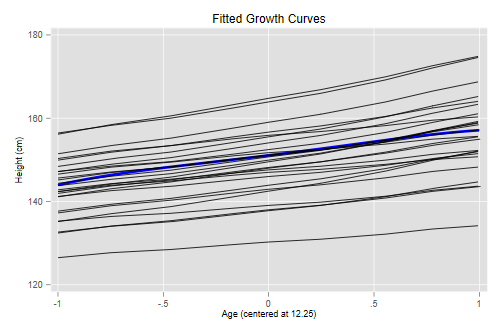```> oxboys <- mutate(oxboys, fv = predict(s2))
> ggplot(group_by(oxboys, id), aes(age, fv, group=id)) +
+   geom_point(aes(age, height), color="#c0c0c0") + geom_line() +
+   ggtitle("Fitted Growth Curves")
> ggsave("oxboysfitsr.png", width = 500/72, height = 400/72, dpi = 72)
```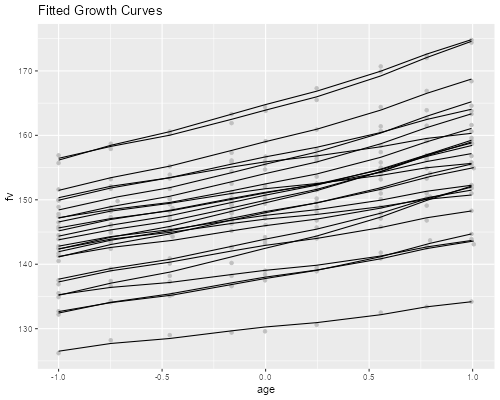The curves reflect substantial variation in growth curves across children, with large differences in average height.

The coefficient of the cosine term or amplitude is estimated at -0.236. We can plot the estimated curve for the range of the data

```. twoway function y = _b[sc] * cos(_pi * x/6), range(0.84 9.36) ///
>     title(Seasonal Component) xt(Season) yt(cm)

. graph export oxboysseasons.png, width(500) replace
file oxboysseasons.png saved as PNG format
```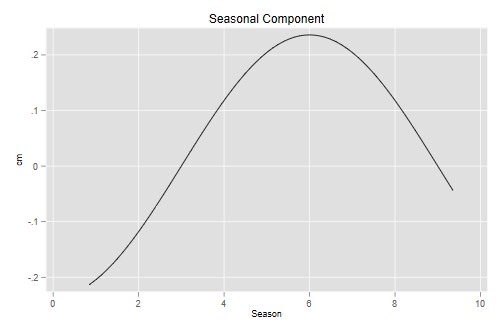```> x <- seq(0.84, 9.36, 0.01)
> seas <- data.frame(season = x,
+     cm = fixef(s2)["sc"] * cos(pi*x/6))
> ggplot(seas, aes(season, cm)) + geom_line() +
+     scale_x_continuous(breaks=seq(2,8,2)) +
+     ggtitle("Seasonal Component")
> ggsave("oxboysseasonsr.png", width = 500/72, height = 400/72, dpi = 72)
```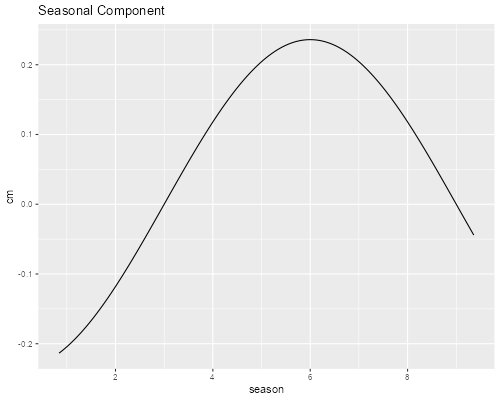The estimates show that boys grow about half a centimeter more in the summer than in the winter.

For residuals with a gap ot t the serial correlation is ρ(t) = exp{-γ t}. We estimate γ as 6.91. Both Stata and R report ρ(1) = 0.001, but we can solve for γ. The plot below shows the correlation function in (0,1) but we label the axis in months

```. scalar rho = invlogit(_b[r_logitr1:_cons])

. scalar gamma = -log(rho)

. mata exp(-st_numscalar("gamma"):*(0.25,.50,.75))
1             2             3
┌───────────────────────────────────────────┐
1 │  .1778326191   .0316244404   .0056238571  │
└───────────────────────────────────────────┘

. twoway function y=exp(-6.907647*x/12), range(0 12) ///
>     title(Serial Correlation of Residuals) ///
>     yt("r(t)") xt(t (in months)) xlab(0(3)12)

. graph export oxboysrho.png, width(500) replace
file oxboysrho.png saved as PNG format
```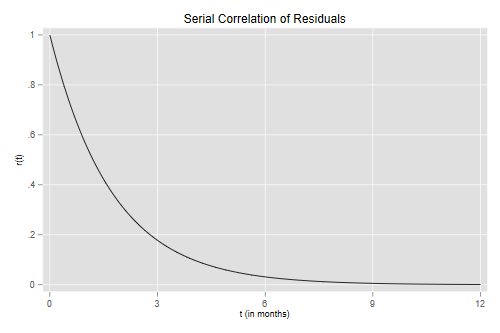```> gamma <- -6.907647
> exp(gamma * 1:3/4)
 0.17783275 0.03162449 0.00562387
> months <- seq(0, 12, 0.1)
> serial = data.frame(months = months,
+     rho = exp(gamma * months/12))
> ggplot(serial, aes(months, rho)) + geom_line() +
+     scale_x_continuous(breaks = seq(0,12,2)) +
+     ggtitle("Serial Correlation of Residuals")
> ggsave("oxboysrhor.png", width = 500/72, height = 400/72, dpi = 72)
```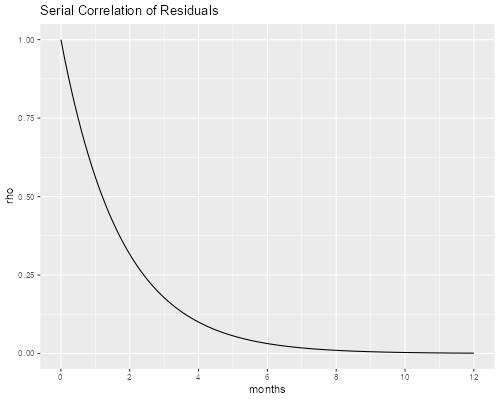The correlation between residuals is 0.178 after 3 months and falls to 0.032 after 6 months.

### Correlation Between Outcomes

The serial correlation is just part of the correlation between outcomes in the same child. Let us calculate the correlation between heights at ages 11.25 and 11.5 for child i.

Those outcomes involve the random effects (ai, bi, ci, ei1, ei2)’, which have variance-covariance matrix V.

The random part of the heights at those ages is a linear combination of those random effects with coefficients C given below, so their variance-covariance is given by C V C’:

```. estat recov // extract variance-covariance of random effects

Random-effects covariance matrix for level id

│       age       age2      _cons
─────────────+─────────────────────────────────
age │  2.680292
age2 │  .8524706   .5745081
_cons │  7.930183   1.479247   61.47597

. mata:
───────────────────────────────────────────────── mata (type end to exit) ──────
:   V = st_matrix("r(cov)")

:   b = st_matrix("e(b)")

:   s2e = exp(2*b)             // error variance

:   rho = invlogit(b)^0.25  // serial correlation

:   E =  (s2e, s2e * rho \ s2e * rho, s2e)

:   Z = J(3,2,0)

:   V = V, Z \ Z', E

:   C = (-1, 1, 1, 1, 0 \ -0.75, 0.75^2, 1, 0, 1)

:   A = C * V * C'

:   D = diag(1:/sqrt(diagonal(A)))

:   D * A * D
[symmetric]
1             2
┌─────────────────────────────┐
1 │            1                │
2 │  .9955685415             1  │
└─────────────────────────────┘

: end
────────────────────────────────────────────────────────────────────────────────
```
```> V <- getVarCov(s2)
> s2e <- s2\$sigma^2
> rho <- 0.1778327566
> E <- matrix( c(s2e, s2e * rho, s2e * rho, s2e), 2, 2)
> zero <- matrix(0, 3, 2)
> V <- rbind(cbind(V, zero), cbind(t(zero),E))
> # intercept is first
> C <- matrix(c(1, -1, 1, 1, 0,  1, -0.75, 0.75^2, 0, 1), 2, 5, byrow = TRUE)
> A <- C %*% V %*% t(C)
> D <- diag(1/sqrt(diag(A)))
> D %*% A %*% D
[,1]      [,2]
[1,] 1.0000000 0.9955686
[2,] 0.9955686 1.0000000
```

This leads to a correlation of 0.996. The observed correlation, which is easy to obtain if we recast the data in wide format, is also 0.996.

### The Deviance Table

We now calculate reductions in deviance starting from the population average model, letting the intercept, slope and curvature be random, and finally allowing for serial correlation

```. quietly xtmixed height age age2 age3 age4 sc, mle

. estimates store ols

. quietly xtmixed height age age2 age3 age4 sc || id: , mle

. estimates store ri

. lrtest ols .

Likelihood-ratio test
Assumption: ols nested within ri

LR chi2(1) = 712.33
Prob > chi2 = 0.0000

Note: The reported degrees of freedom assumes the null hypothesis is not on
the boundary of the parameter space. If this is not true, then the
reported test is conservative.

. quietly xtmixed height age age2 age3 age4 sc || id: age ///
>   , mle covariance(unstructured)

. estimates store rs

. lrtest ri .

Likelihood-ratio test
Assumption: ri nested within rs

LR chi2(2) = 260.73
Prob > chi2 = 0.0000

Note: The reported degrees of freedom assumes the null hypothesis is not on
the boundary of the parameter space. If this is not true, then the
reported test is conservative.

. quietly xtmixed height age age2 age3 age4 sc || id: age age2 ///
>   , mle covariance(unstructured)

. estimates store rq

. lrtest rs .

Likelihood-ratio test
Assumption: rs nested within rq

LR chi2(3) =  52.93
Prob > chi2 = 0.0000

Note: The reported degrees of freedom assumes the null hypothesis is not on
the boundary of the parameter space. If this is not true, then the
reported test is conservative.

. quietly xtmixed height age age2 age3 age4 sc || id: age age2 ///
>   , mle covariance(unstructured) residuals(exponential, t(age))

. lrtest rq .

Likelihood-ratio test
Assumption: rq nested within .

LR chi2(1) =   2.06
Prob > chi2 = 0.1512
```
```> mf <- height ~ age + age2 + age3 + age4 + sc
> m0 <- lm(mf, data = oxboys)
> m1 <- lme(mf, random = ~ 1 | id, data = oxboys, method = "ML")
> cat("0 vs 1", "L.Ratio = ", 2*(logLik(m1) - logLik(m0)),"\n")
0 vs 1 L.Ratio =  712.3291
> m2 <- update(m1, random = ~ age | id, method="ML")
> m3 <- update(m2, random = ~ age + age2 | id)
> m4 <- update(m3, correlation = corCAR1(form = ~ age | id))
> anova(m1, m2, m3, m4)
Model df      AIC      BIC    logLik   Test   L.Ratio p-value
m1     1  8 943.2427 970.8853 -463.6213
m2     2 10 686.5144 721.0677 -333.2572 1 vs 2 260.72824  <.0001
m3     3 13 639.5809 684.5001 -306.7905 2 vs 3  52.93350  <.0001
m4     4 14 639.5205 687.8950 -305.7602 3 vs 4   2.06045  0.1512
```

All tests are on a boundary of the parameter space and thus are conservative. They are all significant except for serial correlation.

